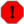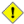## Name

= — Returns `TRUE` if A's bounding box is the same as B's (uses float4 boxes).

## Synopsis

`boolean =(` geometry A , geometry B `)`;

`boolean =(` geography A , geography B `)`;

## Description

The `=` operator returns `TRUE` if the bounding box of geometry/geography A is the same as the bounding box of geometry/geography B. PostgreSQL uses the =, <, and > operators defined for geometries to perform internal orderings and comparison of geometries (ie. in a GROUP BY or ORDER BY clause).This is cause for a lot of confusion. When you compare geometryA = geometryB it will return true even when the geometries are clearly different IF their bounding boxes are the same. To check for true equality use ST_OrderingEquals or ST_Equals. Even for points, doing a bounding box check is not sufficient to determine true equality of points since bounding box prior to PostGIS 2.0 are stored as float4.This operand will NOT make use of any indexes that may be available on the geometries.This method supports Circular Strings and Curves

## Examples

```SELECT 'LINESTRING(0 0, 0 1, 1 0)'::geometry = 'LINESTRING(1 1, 0 0)'::geometry;
?column?
----------
t
(1 row)

SELECT ST_AsText(column1)
FROM ( VALUES
('LINESTRING(0 0, 1 1)'::geometry),
('LINESTRING(1 1, 0 0)'::geometry)) AS foo;
st_astext
---------------------
LINESTRING(0 0,1 1)
LINESTRING(1 1,0 0)
(2 rows)

-- Note: the GROUP BY uses the "=" to compare for geometry equivalency.
SELECT ST_AsText(column1)
FROM ( VALUES
('LINESTRING(0 0, 1 1)'::geometry),
('LINESTRING(1 1, 0 0)'::geometry)) AS foo
GROUP BY column1;
st_astext
---------------------
LINESTRING(0 0,1 1)
(1 row)
-- NOTE: Although the points are different, the float4 boxes are the same
-- In versions 2.0+ and after, this will return false since 2.0+ switched
-- to store double-precision (float8) bounding boxes instead of float4 (used in 1.5 and prior) --
SELECT ST_GeomFromText('POINT(1707296.37 4820536.77)') =
ST_GeomFromText('POINT(1707296.27 4820536.87)') As pt_intersect;
--pt_intersect --
t```